## Friday, June 1, 2018

### MCQ Review of 9708/32 of Summer 2016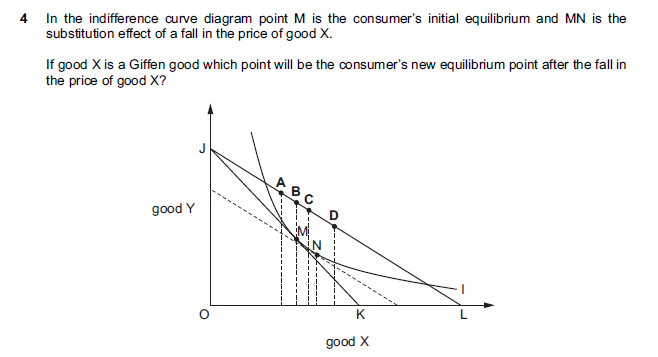When there is a fall in the price of a good, its quantity demanded will usually increase. For the case of normal goods, both substitution and income effects are positive. As for inferior goods, substitution effect is positive but income effect is negative. However, since substitution effect is stronger, there will still be an overall increase in quantity demanded. Giffen goods e.g. mud cakes in Haiti have positive substitution effect but negative income effect (just like the case of inferior goods). However, because negative income effect is more dominant, this results in an overall decline in quantity demanded when there is a fall in its price. M to N is positive substitution effect. N to A is negative income effect. Answer is therefore A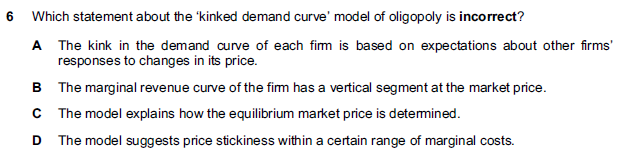A, B and D are correct. The only incorrect option is C. One biggest limitation of the kinked demand curve theory is that it does not explicitly explain how oligopoly firms 'agree'/ ended up setting the price and output at the kinked part of the demand/ AR curve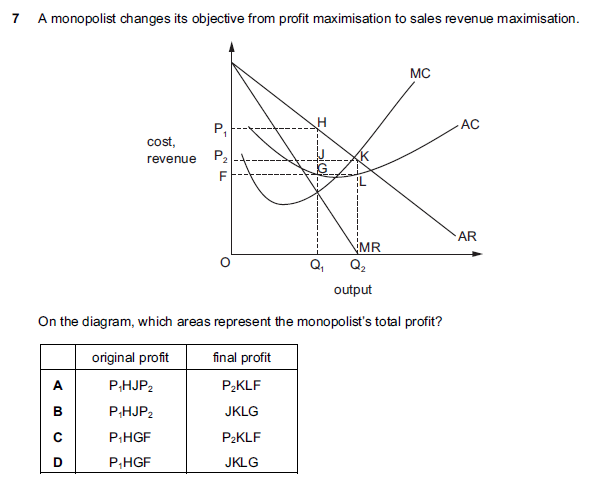Profit maximisation happens when output is at the level where MC = MR. What this means is that, when the gradient of the TC curve and TR curve is equal, the gap between the TC and TR curves will be at its greatest and hence profits are said to be maximised. Revenue maximisation is when output settles where MR = 0. When TR curve reaches its peak/ the turning point, its gradient which is MR will be equivalent to 0. This is why we say MR = 0. Sales maximisation is the highest level of output that a firm will sell without incurring a loss. Therefore it will sell up to TC = TR where normal profit is earned. But if TC/Q = TR/Q, we will get AC = AR, hence the condition of sales maximisation (don't get them mixed up again!). Initial profit area = P1HGF (MC = MR, must minus cost). New profit area = P2KLF (MR = 0, again must minus cost). Answer is C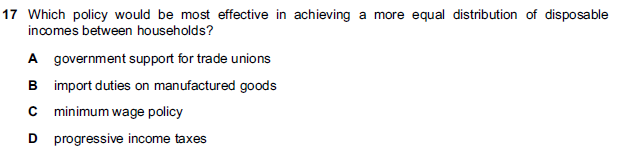It cannot be A. Rising influence of trade unions may create labour market inflexibility. If firms find it difficult to fire, they will not hire as well. Unemployment may persist into the long-run. B is incorrect either. Import duties imposed may most likely cause trade retaliations. Manufacturing jobs will be lost and it is the low income people that suffer the most. C will cause some jobs to be lost too. The more sensible and effective way to create income equality is through progressive income taxes. Answer is D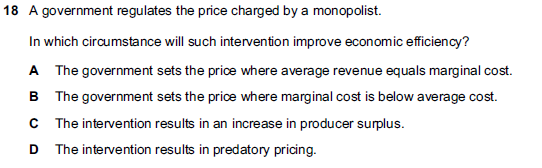In microeconomics, economics efficiency is attained when MC = AC (producing at the lowest cost possible) and MC = AR (marginal cost pricing, since AR = P). Answer is A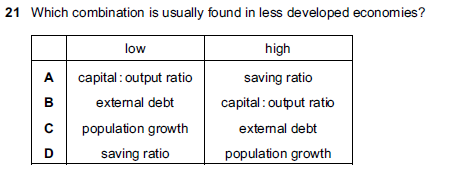A is incorrect. In poor countries, people spend most of their income onto food and other basic necessities. They barely save. So savings ratio is low. B is incorrect too. External debt is usually very high, which explains why many of them are placed under the HIPC (Highly Indebted Poor Countries) initiative. C is obviously wrong. Population growth is high. Answer is D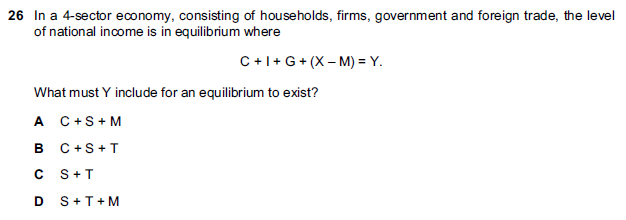The answer is B. For equilibrium to exist, injections must be equal to leakages. The initial equation is {C + I + G + (X - M)} = Y. If you equate it to {C + S + T}, you would get {C + I + G + (X - M)} = {C + S + T}. By cancelling out the C on both sides and bringing M from left to the right, you would get injections = withdrawal